# Tabular Cumulative Summation (CUSUM) Chart

Control charts have been around for nearly 100 years and were first created by Dr. Walter Shewhart at the Western Electric Company in the 1920's. Published in 1931, his ground-breaking book, Economic Control of Quality of Manufactured Product, set the standard for modern statistical quality control methods.

Today, many quality control texts have been written which highlight modifications to Shewhart's techniques. Most of these enhancements have been born of the need to better support modern manufacturing methods and challenges. One such chart is the Tabular Cumulative Summation chart, or simply, the CUSUM chart.

### What is a CUSUM chart?

Like control charts, CUSUM charts are used to plot data in time-series. The charts are meant to alert users to significant changes in process performance. CUSUM charts do not plot raw data values, averages, ranges or standard deviations. Instead, plot points on a CUSUM chart are data values roughly representative of the cumulatively summed, subgroup-to-subgroup deviations from a specified target or production mean. The primary advantage of the tabular CUSUM chart is that the chart is more sensitive to small changes in the mean, especially when compared with IX-MR control charts.

### Why Use a CUSUM Chart?

Basically, it boils down to sensitivity. and Range and and S control charts are commonly understood, powerful process control tools. Their power lies in plotting average values which become more normally distributed as the subgroup size, n, increases. The larger the subgroup size, the more sensitive charts become in detecting significant shifts in the mean. Increasing control chart sensitivity is as simple as increasing subgroup size.

But not everyone has the luxury of creating large subgroups. Consider manufacturing organizations who specialize in short production runs or who rely on destructive testing to quantify product quality. In these cases, very little data is available, or it is cost-prohibitive to collect. Therefore, large subgroups are not an option.

If subgrouping cannot be leveraged, then an Individual X and Moving Range (IX-MR) control chart might seem like a good alternative to and Range control charts. However, because plotted points on IX charts are individual data values (not averages), the IX values are less sensitive to small changes in the overall mean, especially when compared with charts.

Another reason CUSUM charts are popular is their ability to mitigate risk. For example, consider companies who tempt catastrophe when critical data values stray too far away from production targets. These organizations cannot afford to wait until plot point falls outside +/-3σ control limits before generating an alarm. Instead, they need greater sensitivity to small process changes.

Each of the situations above indicates why CUSUM charts are useful. When small changes in the mean (1 - 1.5σ) must be identified and when very little data is available for process control purposes, the CUSUM chart is an excellent solution. As such, the CUSUM chart is popular in the aerospace, metallurgical, chemical and continuous processing industries.

### How Are CUSUM Charts Created?

When compared with traditional control charts, the tabular CUSUM chart is unique, and certain parameters and statistics must be used in its creation.

 Parameter Description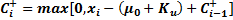The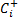plot point is used exclusively for plotting the upper line on the tabular CUSUM chart. The upper line is used for identifying changes in the process above the stated target value,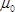.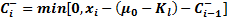The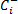point is used exclusively for plotting the lower line on the tabular CUSUM chart. The lower line is used for identifying changes in the process below the chosen target value,.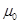Process Mean or Target value.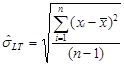Sample standard deviation, or Long Term (LT) standard deviation.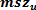The positive shift in Process Mean that the CUSUM chart is meant to detect, in standard deviation units. Typically between 0.5σ and 1.5σ.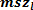The negative shift in Process Mean that the CUSUM chart is meant to detect, in standard deviation units. Typically between 0.5σ and 1.5σ.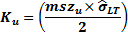The "Upper Reference" value specified for plotted Positive CUSUM line. Positive CUSUM values are accumulated only when the deviation from the target value exceeds specified K value. K value is half the magnitude of the mean shift value to detect.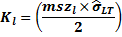The "Lower Reference" value specified for plotted Negative CUSUM line. Negative CUSUM values are accumulated only when the deviation from the target value exceeds specified K value. K value is half the magnitude of the mean shift value to detect.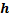Decision Parameter. Factor for determining Decision Interval, H. Generally, h is defined as 5, although sometimes 4 is utilized.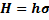H is the "Decision Interval," which acts as a control limit.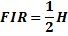Optional. Fast Initial Response or "head start" is typically half of H and is treated as the initial CUSUM value when no data exists.

Figure 1 Table of parameters and statistics necessary to construct the tabular CUSUM chart.

### A Tabular CUSUM Example

A steel manufacturer makes large I-beams for the construction industry. I-beams are large, expensive to manufacture and are made infrequently. One of the critical features, the "A-Dimension," must be closely controlled and is difficult to measure.

If the A-Dimension average sustains a shift of more than 1σ above its overall target, then the strength of the product could be compromised and product could be scrapped, resulting in significant financial loss. To prevent these issues, the company wants line operators to be notified when a shift of at least one σ has occurred in the A-Dimension's average.

Historically, the A-Dimension has mean value of close to 50.00 and a historical standard deviation of around 0.70. Data has been collected for the last 28 A-Dimension values. They are found in the table below. The asterisks found next to subgroups 21-28 indicate that the mean changed from 50.00 to 50.75 - approximately a one σ increase.

 Subgroup # A-Dimension Subgroup # A-Dimension 1 50.453 15 49.895 2 50.682 16 50.014 3 49.686 17 49.373 4 49.572 18 50.523 5 51.333 19 51.111 6 50.280 20 50.044 7 49.240 21* 51.601 8 50.478 22* 50.479 9 49.263 23* 49.089 10 50.046 24* 50.632 11 49.540 25* 50.373 12 49.270 26* 51.682 13 50.316 27* 50.521 14 49.512 28* 51.639

Figure 2 Table of A-Dimension data showing an increase of one σ in the last 8 subgroups.

A traditional IX-MR control chart of the A-Dimension data is found in Figure 3. The blue vertical line indicates the beginning of a sustained increase of 1σ in the mean A-Dimension value.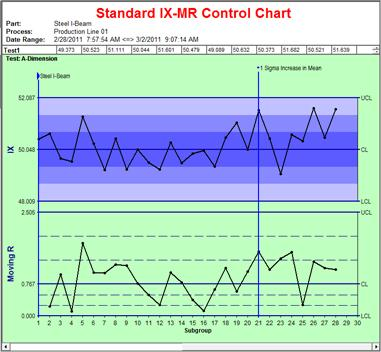Figure 3 IX-MR control chart of A-Dimension data.

Although there is a sustained 1σ increase in the A-Dimension for the final 8 values on the IX-MR control chart, no data values fall outside control limits. This shift is difficult for the IX chart to pick up because of the small subgroup size and the chart's inherent inability to sense small changes in the mean.

To demonstrate the advantages of the tabular CUSUM chart, the same data found in the table from Figure 2 will be used in the following example.

#### Creating the Tabular CUSUM chart

These are several steps for creating a tabular CUSUM chart:

1. The data from Figure 2 must be converted and plotted into two different CUSUM plot points:

a.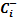values indicating the cumulative summation of values below the target
b.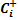values indicating the cumulative summation of values above the target

1. Identification of the target,2. Selection of the positive shift in the mean to detect,3. Selection of the negative shift in the mean to detect,4. Selection of Decision parameter, H

First, the CUSUM plot point values for the steel I-beam example are calculated and found in Figure 4.

 Subgroup # A-Dimension CUSUM Plot Point UpperCUSUM Plot Point Lower1 50.453 0.065 0.000 2 50.682 0.359 0.000 3 49.686 0.000 -0.022 4 49.572 0.000 -0.158 5 51.333 0.946 0.000 6 50.280 0.838 0.000 7 49.240 0.000 -0.468 8 50.478 0.091 0.000 9 49.263 0.000 -0.445 10 50.046 0.000 -0.107 11 49.540 0.000 -0.275 12 49.270 0.000 -0.714 13 50.316 0.000 -0.106 14 49.512 0.000 -0.301 15 49.895 0.000 -0.114 16 50.014 0.000 0.000 17 49.373 0.000 -0.335 18 50.523 0.135 0.000 19 51.111 0.858 0.000 20 50.044 0.515 0.000 21* 51.601 1.728 0.000 22* 50.479 1.819 0.000 23* 49.089 0.520 -0.619 24* 50.632 0.764 0.000 25* 50.373 0.749 0.000 26* 51.682 2.044 0.000 27* 50.521 2.177 0.000 28* 51.639 3.428 0.000

Figure 4 CUSUM plot points calculated for both upper and lower CUSUM lines.

#### Plotted Points

Following are the assumptions and calculations necessary for completing the CUSUM chart for the steel I-beam example:

1.= Target Value = Actual Process mean = 50.048
2.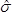= Estimated Standard Deviation = 0.6796
3.= Positive mean shift to detect (z) = 1.0σ
4.= Negative mean shift to detect (z) = 1.0σ
5.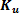= Upper Reference =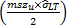=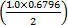= 0.3398
6.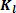= Lower Reference =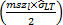=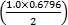= 0.3398
7. h = Decision Parameter = 5
8. H = Decision Interval = h= 5 ×= 5 × 0.6796 = 3.398

Begin creating the CUSUM chart by calculating the Upper and Lower CUSUM plot points using this equation: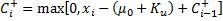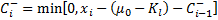Each plot point is the larger of either one of two results:

1. Zero or
2. The sum ofand K, subtracted from the current subgroup data value, xi, then added to the previousplot point (denoted by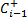).

Examples of Plot Point Calculations for the Upper and Lower CUSUM Line:

 Upper CUSUM Calculations:Lower CUSUM Calculations: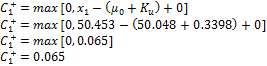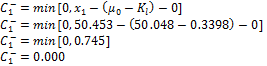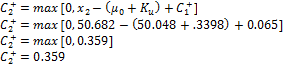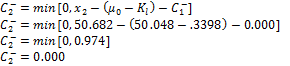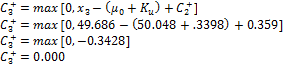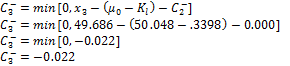Figure 5 Calculated plot points for the first 3 subgroups on the CUSUM chart.

Once both the Upper and Lower CUSUM values have been calculated, they are plotted on the same chart (see Figure 6).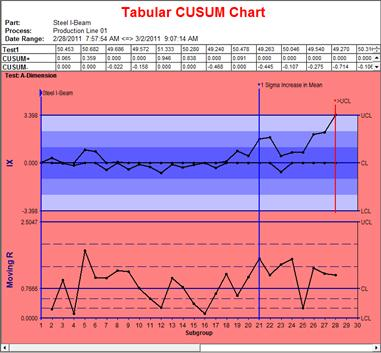Figure 6 Steel I-Beam A-Dimension data plotted on a tabular CUSUM chart.

#### Tabular CUSUM Chart Interpretation

With the notable exception of the dual plot point lines on the upper chart, the tabular CUSUM chart looks much like a Shewhart control chart. And that is part of its attraction. The two different plotted lines allow the steel manufacturer to simultaneously view cumulative summations for both above and below the Target. While the plot points are calculated on the top chart, the CUSUM calculations have no effect on the Moving Range plot points in the chart below. If there is not a significant shift in the mean, CUSUM plot points tend to gather around the center line, zero. This is true for the first 20 plot points.

Recall that the first twenty subgroups have a mean of 50.048 and an estimated standard deviation,, of .6796. From the 21st through the 28th subgroup, the mean has changed to 50.75. This is an increase of a little more than 1σ in the A-Dimension mean.

The steel company was especially concerned with an increase in the mean value above the Target. Unlike the IX-MR control chart in Figure 3, the CUSUM chart has triggered an alarm, indicating an increase in the mean of more than 1σ. Collectively, plot points after the 20th subgroup fall farther above the centerline when compared with data values prior to the change. This is further evidence of a sustained increase in the average.

Additionally, the last 4 plot points steadily increase until the last plot point falls outside the upper control limit (H value = 5= 5(.6796) = 3.398). Unlike independent plot points on an IX-MR control chart, each CUSUM plot point is influenced by previously plotted values. Not so with IX-MR charts. This fact helps tabular CUSUM charts provide additional sensitivity to small changes in the mean.

Need help?
​For further information contact GetInTouch@infinityqs.com
Toll Free: 1.800.772.7978​

### Take the first step from quality to excellence

Take the Next Steps